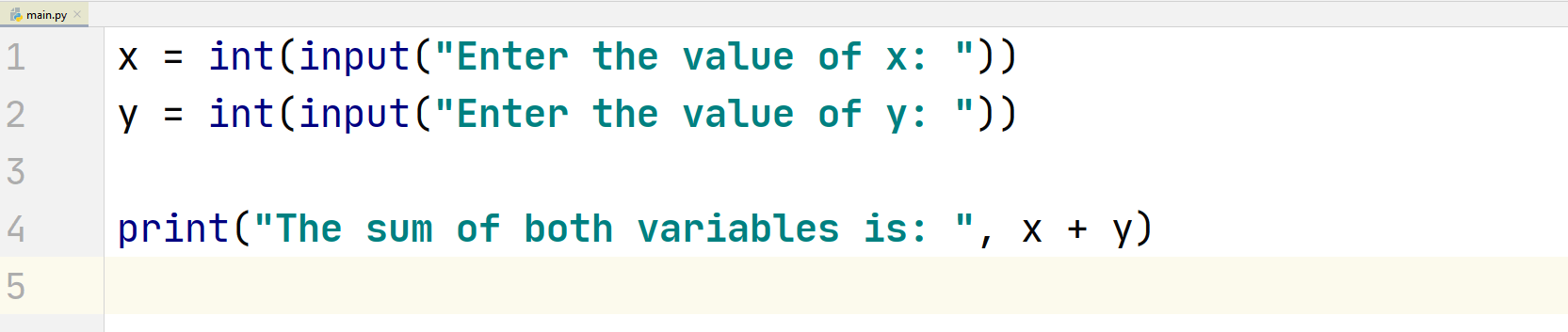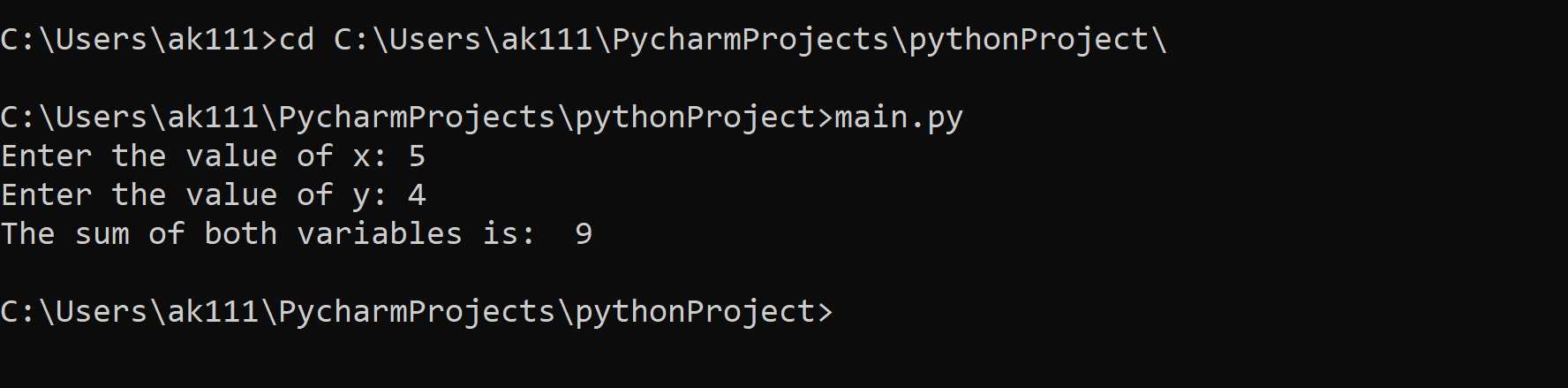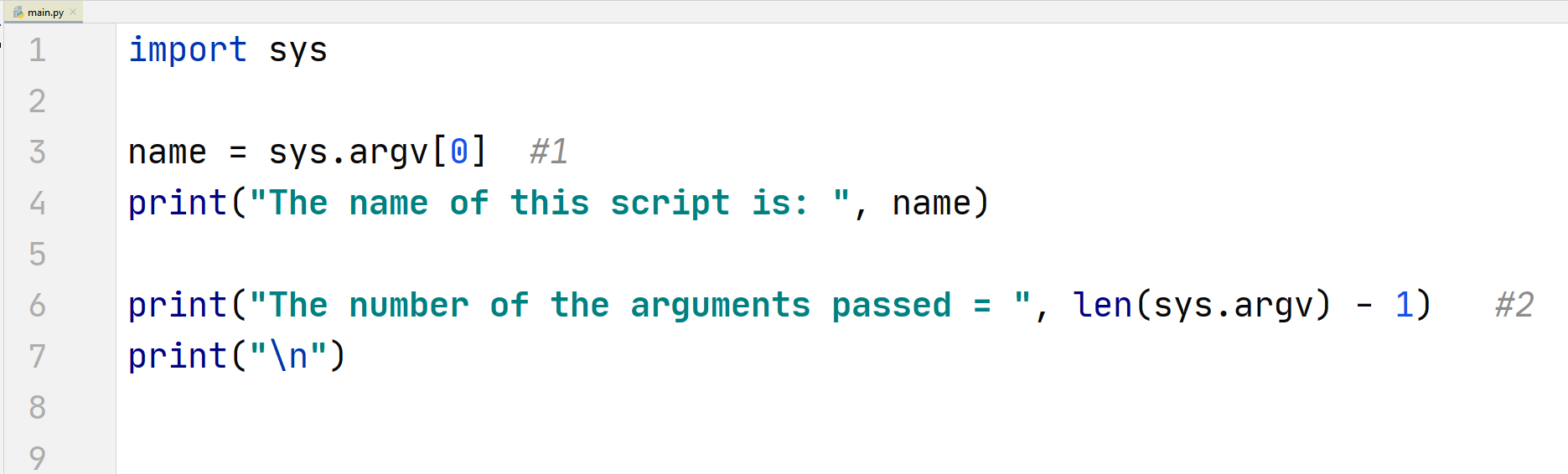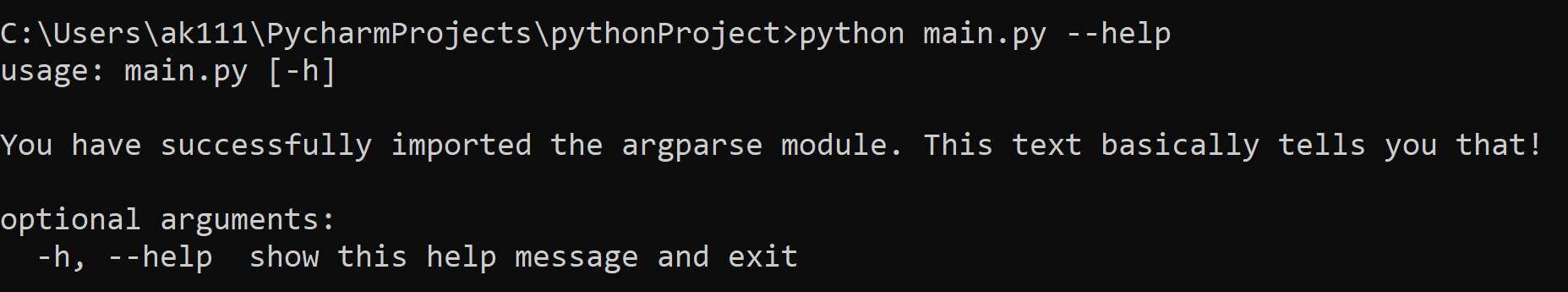# Python Command Line

## Python Command Line

You can run a python script in a command line. The arguments that you give in the command line that follow the script name in Python are called Command Line Arguments.

There are different ways you can interact with the command line arguments in Python. The most common of them are:

• sys module
• getopt module
• argparse module

Each of them have their advantages and disadvantages. To use these arguments, first let us see how to run a Python script in a command prompt.

## Python Script in Command Line

First, let’s write some code in our Python script.

## ExampleOur program is simple, it takes input of two numbers and adds them. Now, save the program and navigate to the directory where you have saved this program.

To navigate to the directories, in your operating software (Windows/Ubuntu/MacOs) you can use the cd command followed by the path of the directory.

In Windows, you can follow an easier approach, just simply press  shift + right click in the folder where the script is saved and select the open command prompt here option.

After, opening the command line program in your operating software, write the following command:

\$ python “name of the script”

and hit Enter. The script will ask you to enter the values of the variables, enter them, now it should display the output.

## Example## Python Command Line Arguments

We will have a look at the methods that you can utilize to use the command line arguments but first we will have to see what functions each module provides us and how we can use those functions to interact with the command line.

## sys module

sys module is one of the original modules that come with Python pre-installed from the beginning. It is used to make us able to manipulate the various parts of the Python environment.

These are some points that you need to know about this module to use command line arguments in Python.

• sys has a variable argv that contains a list of command line arguments in an easy to access list form.

• Each element of the list represents one argument except the first element, and by using the len(sys.argv), you can see the number of arguments present in the list.

• The first element, i.e. sys.argv returns the name of the Python script that is running.

• When you separate arguments in the command line, a whitespace is used instead of a comma separation to tell Python that the arguments are different.

To use this variable, we will have to import the sys module.

## Example 1 of sys moduleOutput of the above python script in command promptsys module code explanation:

1. We store the name of the script in the name variable and print it out.

2. Next, using the len() function to get the size of the sys.argv list. By subtracting 1 from the list size, we get the actual number of the arguments passed because the first element in the list is the name of the script

But arguments are of no use unless we do something with them, right? So, let’s see how to use the arguments passed.

We are going to write a program that multiplies all of the arguments together.

## Example 2 of sys module​Output of the above python script in command promptsys module code explanation:

1. By using [1:], we access the part of the list that contains the argument values.
2. We iterate through the arguments passed using the for loop. The list elements are stored as strings, to convert them into integers, we use the int() function and do our multiplication.

## getopt module

getopt module uses the getopt() function that provides a selection between long and short options with a value assignment. The sys module acts like a dependency for this module, which means that the getopt module will not be of any use without the sys module.

It is a similar module to the C language’s getopt function. To use this module in Python, you will need to remove the first element in the sys.argv variable list.

Before using this module, let us understand the syntax of this function:

Syntax:

getopt.getopt(args, options, [long_options])

Here what each term means:

• args: This is the list we will be passing that will contain the arguments.

• options/short_options: These are the operations that we can write in the command line and can be recognised by our script. The parameters “option” are also called short_options.
The options that can accept arguments are followed by a colon (:)

• long_options: Similar to the option parameter but they have a long name that is associated with their short option counterpart.
The long_options that takes arguments are followed by an equal sign (=)

To understand the syntax better, consider this for an example:

## ExampleOutput of the above python script in command promptUnderstanding the program:

1. Using indexing [1:], we extract the arguments from the sys.argv list and store it in the argument_list variable.

2. We define the short options as “vho:”. In this “vho:” term, each letter acts as a different entity, and the colon (:) operator that is used to accept arguments is only associated with the letter “o”.

3. Next we define our long options’ list, this list will contain all of the long options that can be used in place of the short options. It is recommended to use appropriate naming for the long option list as it may get difficult to memorize.

4. We create two variable args and val. The args variable is equal to the arguments that we are using for the getopt module and the val is equal to the values that are present in the argument list of the sys.argv variable.

5. Now, we create a for loop that iterates through the args list variable. And, then we create appropriate if statements that get triggered when specific values are passed. For short options, a single hyphen “-” is used, and for long options, double hyphens “–” are used.

What if there is a better option than these two methods to pass command line arguments? Yes, there is a better method.

## argparse module

argparse module provides features like, help message, data type specification, positional arguments, etc. Therefore it is considered to be a better option than the two described above.

argparse module comes in-built since Python 3.2, so if you are using an older version of Python, then you will have to update it.

Basically, this module shortens the amount of work done by you, unlike the above two modules that leave most of the stuff into your hands.

Let’s import the module and start using it.

## Example 1 of argparse moduleOutput of the above python script in command promptThe program above is a simple program that is just used to initialize the parser that takes some input data and builds an output structure.

Now, let’s see how you can include some text that will get printed when you use the help command for the argparse module.

## Example 2 of argparse moduleOutput of the above python script in command promptYou need to pass the string in the ArgumentParser to get the string initialized. When you use the –help or -h command, this string gets printed on the screen.

Now, let us add an optional argument other than -h or –help. We will use this program to print out the sum of two numbers.

## Example 3 of argparse moduleOutput of the above python script in command promptWe store the parser arguments in a variable named argument and if the value of argument.sum i.e. our command is true, then we ask the user to enter two numbers and print their sum.